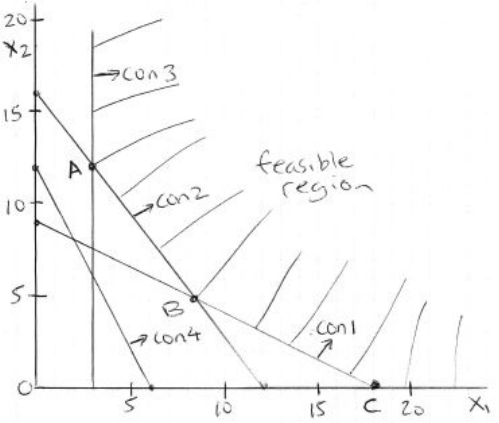Study Guides (380,000)
CA (150,000)
UTSC (10,000)
Midterm

# MGOC10H3 Study Guide - Midterm Guide: White Meat, CutletExam

Department
Management
Course Code
MGOC10H3
Professor
Vinh Quan
Study Guide
Midterm

This preview shows half of the first page. to view the full 3 pages of the document.1
Department of Management, UTSC
MGOC10 Analysis for Decision Making Midterm Solution
Q1.
(a) a = 0, b = 0, c = 37.5, d = 2.65625
(b)
7 3.125 ≤ coeff C 7 + .875 3.875 ≤ coeff C 7.875
New Z = 242.6562 - (7-5)*(31.25)= 180.1562
(c)
250 51.08108 ≤ RHS R2 250 + 24.28571 get approx. 198.91 ≤ RHS R2 274.29
Dual price R2 = -0.109375 means for each unit increase in RHS, Z worst off by -0.109375.
So new Z= 242.6562 - 0.109375 (20) = 240.4687
Q2.
Let Xt = units ordered in quarter t = 12,3,4, It = inventory at end of quarter t= 1,2,3,4
Min Z = 20X1 + 22X2 + 24X3 + 26X4 + 25(I1 + I2 + I3 + I4)
I1 = 80 + X1 300
I2 = I1 + X2 400
I3 = I2 + X3 450
I4 = I3 + X4 250
X1 ≤ 400, X2 ≤ 400, X3 ≤ 400, X4 ≤ 400
I1 ≤ 100, I2 ≤ 100, I3 ≤ 100, I4 ≤ 100
Q1: 350 25 ≤ X1 ≤ 350 + 35 → 325 ≤ X1 ≤ 385 → 325 ≤ X1 and X1 ≤ 385
Q2: X1 25 ≤ X2 ≤ X1 + 35 → X1 - 25 ≤ X2 and X2 ≤ X1 + 35
Q1: 80 15 ≤ I1 ≤ 80 + 15 → 65 ≤ I1 ≤ 95 → 65 ≤ I1 and I1 ≤ 95
Q2: I1 15 ≤ I2 ≤ I1 + 15 → I1 – 15 ≤ I2 and I2 ≤ I1 + 15
All variables ≥ 0
Q3.
5x1 + 10x2 ≥ 90 → (0,9) (18,0)
4x1 + 3x2 ≥ 48 (0,16) (12,0)
0.5x1 ≥ 1.5 → x1 ≥ 3
4x1 + 2x2 ≥ 24 (0,12) (6,0)
If you draw two Z line, you will see optimal Solution is at point B: x1 = 8.4, x2 = 4.8, Z = 31.2
If objective function is Min Z = 2x1 + 5x2, then optimal Solution is at Point C: x1 = 18, x2 = 0, Z = 36
###### You're Reading a Preview

Unlock to view full version